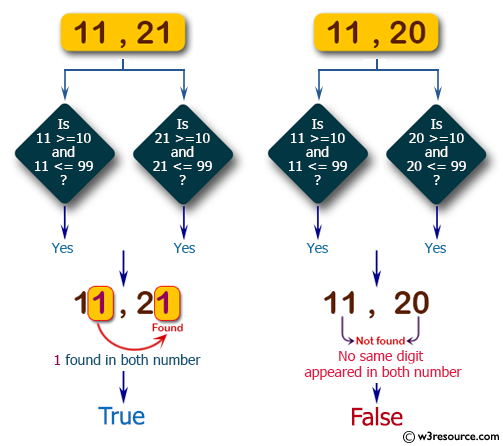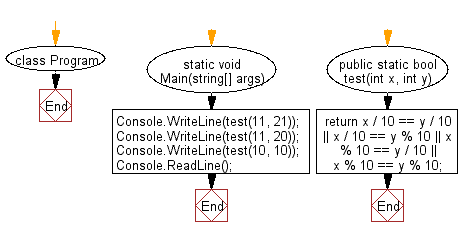﻿ C Sharp - Test 2 given integers, each in the range 10..99# C# Sharp Basic Algorithm Exercises: Check two given integers, each in the range 10..99

## C# Sharp Basic Algorithm: Exercise-53 with Solution

Write a C# Sharp program to check two given integers, each in the range 10..99. Return true if a digit appears in both numbers, such as the 3 in 13 and 33

Pictorial Presentation:Sample Solution:-

C# Sharp Code:

``````using System;
using System.Linq;
namespace exercises
{
class Program
{
static void Main(string[] args)
{
Console.WriteLine(test(11, 21));
Console.WriteLine(test(11, 20));
Console.WriteLine(test(10, 10));
}

public static bool test(int x, int y)
{
return x / 10 == y / 10 || x / 10 == y % 10 || x % 10 == y / 10 || x % 10 == y % 10;
}
}
}
```
```

Sample Output:

```True
False
True```

Flowchart:C# Sharp Code Editor:

Improve this sample solution and post your code through Disqus

What is the difficulty level of this exercise?

Test your Programming skills with w3resource's quiz.

﻿